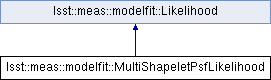lsst::meas::modelfit::MultiShapeletPsfLikelihood Class Reference

Likelihood object used to fit multishapelet models to PSF model images; mostly for internal use by GeneralPsfFitter. More...

#include <GeneralPsfFitter.h>

Inheritance diagram for lsst::meas::modelfit::MultiShapeletPsfLikelihood:## Public Member Functions

MultiShapeletPsfLikelihood (ndarray::Array< Pixel const, 2, 1 > const &image, geom::Point2I const &xy0, std::shared_ptr< Model > model, Scalar sigma, ndarray::Array< Scalar const, 1, 1 > const &fixed)

void computeModelMatrix (ndarray::Array< Pixel, 2,-1 > const &modelMatrix, ndarray::Array< Scalar const, 1, 1 > const &nonlinear, bool doApplyWeights=true) const override
Evaluate the model for the given vector of nonlinear parameters. More...

virtual ~MultiShapeletPsfLikelihood ()

Return the number of data points. More...

int getAmplitudeDim () const
Return the number of linear parameters (columns of the model matrix) More...

int getNonlinearDim () const
Return the number of nonlinear parameters (which parameterize the model matrix) More...

int getFixedDim () const
Return the number of fixed nonlinear parameters (set on Likelihood construction) More...

ndarray::Array< Scalar const, 1, 1 > getFixed () const
Return the vector of fixed nonlinear parameters. More...

ndarray::Array< Pixel const, 1, 1 > getData () const
Return the vector of weighted, scaled data points $$z$$. More...

ndarray::Array< Pixel const, 1, 1 > getUnweightedData () const
Return the vector of unweighted data points $$y$$. More...

ndarray::Array< Pixel const, 1, 1 > getWeights () const
Return the vector of weights $$w$$ applied to data points and model matrix rows. More...

ndarray::Array< Pixel const, 1, 1 > getVariance () const
Return the vector of per-data-point variances. More...

std::shared_ptr< ModelgetModel () const
Return an object that defines the model and its parameters. More...

## Protected Attributes

std::shared_ptr< Model_model

ndarray::Array< Scalar const, 1, 1 > _fixed

ndarray::Array< Pixel, 1, 1 > _data

ndarray::Array< Pixel, 1, 1 > _unweightedData

ndarray::Array< Pixel, 1, 1 > _variance

ndarray::Array< Pixel, 1, 1 > _weights

## Detailed Description

Likelihood object used to fit multishapelet models to PSF model images; mostly for internal use by GeneralPsfFitter.

Definition at line 315 of file GeneralPsfFitter.h.

## ◆ MultiShapeletPsfLikelihood()

 lsst::meas::modelfit::MultiShapeletPsfLikelihood::MultiShapeletPsfLikelihood ( ndarray::Array< Pixel const, 2, 1 > const & image, geom::Point2I const & xy0, std::shared_ptr< Model > model, Scalar sigma, ndarray::Array< Scalar const, 1, 1 > const & fixed )

## ◆ ~MultiShapeletPsfLikelihood()

 virtual lsst::meas::modelfit::MultiShapeletPsfLikelihood::~MultiShapeletPsfLikelihood ( )
virtual

## ◆ computeModelMatrix()

 void lsst::meas::modelfit::MultiShapeletPsfLikelihood::computeModelMatrix ( ndarray::Array< Pixel, 2,-1 > const & modelMatrix, ndarray::Array< Scalar const, 1, 1 > const & nonlinear, bool doApplyWeights = true ) const
overridevirtual

Evaluate the model for the given vector of nonlinear parameters.

Parameters
 [out] modelMatrix The dataDim x amplitudeDim matrix $$B$$ that expresses the model projected in such a way that it can be compared to the data when multiplied by an amplitude vector $$\alpha$$. It should be weighted if the data vector is. The caller is responsible for guaranteeing that the shape of the matrix correct, but implementations should not assume anything about the initial values of the matrix elements. [in] nonlinear Vector of nonlinear parameters at which to evaluate the model. [in] doApplyWeights If False, do not apply the weights to the modelMatrix.

Implements lsst::meas::modelfit::Likelihood.

## ◆ getAmplitudeDim()

 int lsst::meas::modelfit::Likelihood::getAmplitudeDim ( ) const
inlineinherited

Return the number of linear parameters (columns of the model matrix)

Definition at line 77 of file Likelihood.h.

77 { return _model->getAmplitudeDim(); }
std::shared_ptr< Model > _model
Definition: Likelihood.h:147

## ◆ getData()

 ndarray::Array lsst::meas::modelfit::Likelihood::getData ( ) const
inlineinherited

Return the vector of weighted, scaled data points $$z$$.

Definition at line 89 of file Likelihood.h.

89 { return _data; }
ndarray::Array< Pixel, 1, 1 > _data
Definition: Likelihood.h:149

inlineinherited

Return the number of data points.

Definition at line 74 of file Likelihood.h.

74 { return _data.getSize<0>(); }

## ◆ getFixed()

 ndarray::Array lsst::meas::modelfit::Likelihood::getFixed ( ) const
inlineinherited

Return the vector of fixed nonlinear parameters.

Definition at line 86 of file Likelihood.h.

86 { return _fixed; }
ndarray::Array< Scalar const, 1, 1 > _fixed
Definition: Likelihood.h:148

## ◆ getFixedDim()

 int lsst::meas::modelfit::Likelihood::getFixedDim ( ) const
inlineinherited

Return the number of fixed nonlinear parameters (set on Likelihood construction)

Definition at line 83 of file Likelihood.h.

83 { return _model->getFixedDim(); }

## ◆ getModel()

 std::shared_ptr lsst::meas::modelfit::Likelihood::getModel ( ) const
inlineinherited

Return an object that defines the model and its parameters.

Definition at line 105 of file Likelihood.h.

105 { return _model; }

## ◆ getNonlinearDim()

 int lsst::meas::modelfit::Likelihood::getNonlinearDim ( ) const
inlineinherited

Return the number of nonlinear parameters (which parameterize the model matrix)

Definition at line 80 of file Likelihood.h.

80 { return _model->getNonlinearDim(); }

## ◆ getUnweightedData()

 ndarray::Array lsst::meas::modelfit::Likelihood::getUnweightedData ( ) const
inlineinherited

Return the vector of unweighted data points $$y$$.

Definition at line 92 of file Likelihood.h.

92 { return _unweightedData; }
ndarray::Array< Pixel, 1, 1 > _unweightedData
Definition: Likelihood.h:150

## ◆ getVariance()

 ndarray::Array lsst::meas::modelfit::Likelihood::getVariance ( ) const
inlineinherited

Return the vector of per-data-point variances.

Definition at line 102 of file Likelihood.h.

102 { return _variance; }
ndarray::Array< Pixel, 1, 1 > _variance
Definition: Likelihood.h:151

## ◆ getWeights()

 ndarray::Array lsst::meas::modelfit::Likelihood::getWeights ( ) const
inlineinherited

Return the vector of weights $$w$$ applied to data points and model matrix rows.

Will be an empty array if no weights are applied.

Definition at line 99 of file Likelihood.h.

99 { return _weights; }
ndarray::Array< Pixel, 1, 1 > _weights
Definition: Likelihood.h:152

## ◆ _data

 ndarray::Array lsst::meas::modelfit::Likelihood::_data
protectedinherited

Definition at line 149 of file Likelihood.h.

## ◆ _fixed

 ndarray::Array lsst::meas::modelfit::Likelihood::_fixed
protectedinherited

Definition at line 148 of file Likelihood.h.

## ◆ _model

 std::shared_ptr lsst::meas::modelfit::Likelihood::_model
protectedinherited

Definition at line 147 of file Likelihood.h.

## ◆ _unweightedData

 ndarray::Array lsst::meas::modelfit::Likelihood::_unweightedData
protectedinherited

Definition at line 150 of file Likelihood.h.

## ◆ _variance

 ndarray::Array lsst::meas::modelfit::Likelihood::_variance
protectedinherited

Definition at line 151 of file Likelihood.h.

## ◆ _weights

 ndarray::Array lsst::meas::modelfit::Likelihood::_weights
protectedinherited

Definition at line 152 of file Likelihood.h.

The documentation for this class was generated from the following file:
• /j/snowflake/release/lsstsw/stack/lsst-scipipe-0.7.0/Linux64/meas_modelfit/22.0.1-4-g44f2e3d+9e4ab0f4fa/include/lsst/meas/modelfit/GeneralPsfFitter.h